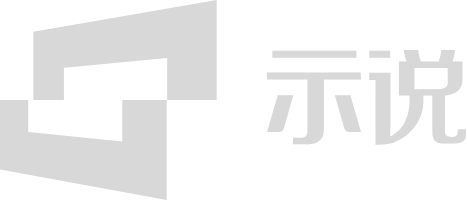HOT10Data Structures and Algorithms--Parallel numerical algorithms# 10Data Structures and Algorithms--Parallel numerical algorithms

The course is mainly about Parallel numerical algorithms.Generally covered Numerical algorithms,Matrix operations,Inner product,Outer product,Matrix vector product,Matrix matrix product,Particle systems,N-body model,Trivial parallelism,Reducing complexity and so on.
0点赞
0收藏
0下载3秒后跳转登录页面